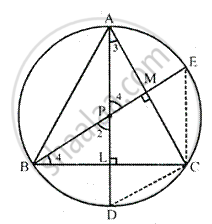Share

# In δ Abc, the Perpendicular from Vertices a and B on Their Opposite Sides Meet (When Produced) the Circumcircle of the Triangle at Points D and E Respectively. Prove That: Arc Cd = Arc Ce - Mathematics

Course

#### Question

In Δ ABC, the perpendicular from vertices A and B on their opposite sides meet (when produced) the circumcircle of the triangle at points D and E respectively. Prove that: arc CD = arc CE

#### Solution

Given: In ΔABC, the perpendiculars from vertices A and B on their opposite sides meet (when produced) the circumcircle of the triangle at points D and E respectively.
To prove: Arc CD = Arc CE
Construction: Join CE and CD
Proof :In ΔAPM and ∠BPL
∠AMP = ∠BLP       [each = 90°]
∠1 = ∠2                 [vertically opposite angles]
ΔAPM ~ ΔBPL       [AA postulate]
∴ Third angle = Third angle
∴ ∠3 = ∠4
∴ Arc which subtends equal angle at the
Circumference of the circle are also equal.
∴ Arc CD = Arc CE

Is there an error in this question or solution?